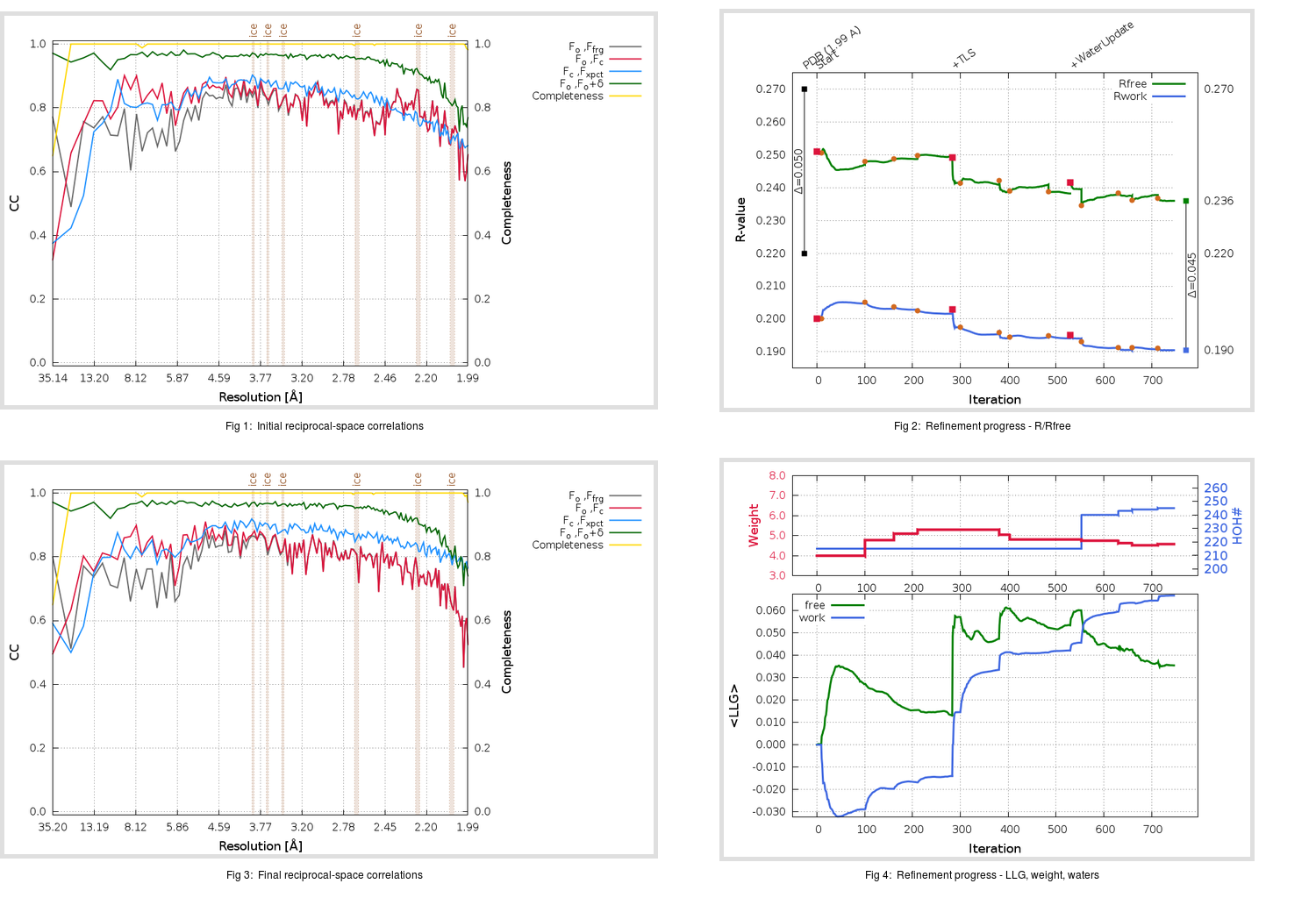Content:

## Deposited

` `
 Date deposited Date data collection Resolution R, Rfree 20110629 20100806 1.99 0.2170 0.2700

Molprobity (CCP4 7.0 version) summary:

```Ramachandran outliers =   0.33 %
favored =  95.39 %
Rotamer outliers      =   4.92 %
C-beta deviations     =     1
Clashscore            =   7.57
RMS(bonds)            =   0.0186
RMS(angles)           =   1.60
MolProbity score      =   2.26
Resolution            =   1.99
R-work                =   0.2170
R-free                =   0.2700
```

```Number of waters      =   215

<B> (all atoms) =   33.19 ( sd =    9.90 ) for       2621 non-hydrogen atoms
<B>   (protein) =   32.39 ( sd =    9.61 ) for       2374 non-hydrogen atoms
<B>     (water) =   41.07 ( sd =    9.53 ) for        215 non-hydrogen atoms
<B>    (others) =   39.65 ( sd =    7.95 ) for         32 non-hydrogen atoms

B min/max       (all non-hydrogen atoms) =   13.75 /   72.43
B min/max   (protein non-hydrogen atoms) =   13.75 /   67.12
B min/max     (water non-hydrogen atoms) =   17.13 /   72.43
B min/max     (other non-hydrogen atoms) =   31.18 /   55.87
```

## BUSTER (re-)refinement

` `

Molprobity (CCP4 7.0 version) summary:

```Ramachandran outliers =   0.00 %
favored =  96.71 %
Rotamer outliers      =   2.27 %
C-beta deviations     =     0
Clashscore            =   2.94
RMS(bonds)            =   0.0114
RMS(angles)           =   1.52
MolProbity score      =   1.56
Resolution            =   1.99
R-work                =   0.1906
R-free                =   0.2362
```

```Number of waters      =   245

<B> (all atoms) =   37.73 ( sd =   11.60 ) for       2651 non-hydrogen atoms
<B>   (protein) =   36.62 ( sd =   11.11 ) for       2374 non-hydrogen atoms
<B>     (water) =   48.16 ( sd =   11.69 ) for        245 non-hydrogen atoms
<B>    (others) =   40.52 ( sd =    4.76 ) for         32 non-hydrogen atoms

B min/max       (all non-hydrogen atoms) =   17.18 /   83.30
B min/max   (protein non-hydrogen atoms) =   18.11 /   83.30
B min/max     (water non-hydrogen atoms) =   17.18 /   77.99
B min/max     (other non-hydrogen atoms) =   33.46 /   49.14
```

Refinement progression:Results:

` `
 File Remark 3SN8_aB_refine.01_03_refine.pdb.gz exact refinement commands are in header 3SN8_aB_refine.01_03_refine.mtz.gz including original deposited data and several re-refinement map coefficients 3SN8_aB_refine.01_03_BUSTER_model.cif.gz including any non-standard compound restraints 3SN8_aB_refine.01_03_BUSTER_refln.cif.gz